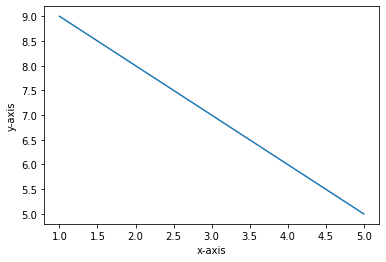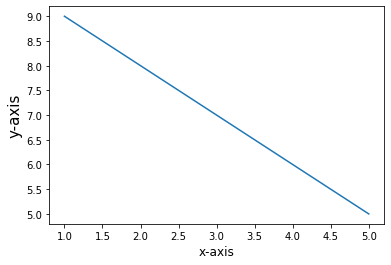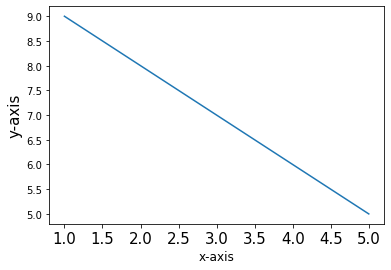Open in App
Not now

# How to change the size of axis labels in Matplotlib?

• Difficulty Level : Easy
• Last Updated : 03 Jan, 2021

Matplotlib is a great data plotting tool. It’s used for visualizing data and also for presenting the data to your team on a presentation or for yourself for future reference. So, while presenting it might happen that the “X-label” and “y-label” are not that visible and for that reason, we might want to change its font size.  So in this article, we are going to see how we can change the size of axis labels in Matplotlib.

Before starting let’s draw a simple plot with matplotlib.

## Python3

 `import` `matplotlib.pyplot as plt`` ` `x ``=` `[``1``, ``2``, ``3``, ``4``, ``5``]``y ``=` `[``9``, ``8``, ``7``, ``6``, ``5``]`` ` `fig, ax ``=` `plt.subplots()``ax.plot(x, y)`` ` `ax.set_xlabel(``'x-axis'``)``ax.set_ylabel(``'y-axis'``)`` ` `plt.show()`

Output### Now we will see how to change the size of the axis labels:

Example 1: Changing both axis label.

If we want to change the font size of the axis labels, we can use the parameter “fontsize” and set it your desired number.

## Python3

 `import` `matplotlib.pyplot as plt`` ` `x ``=` `[``1``, ``2``, ``3``, ``4``, ``5``]``y ``=` `[``9``, ``8``, ``7``, ``6``, ``5``]`` ` `fig, ax ``=` `plt.subplots()``ax.plot(x, y)``ax.plot(x, y)`` ` `ax.set_xlabel(``'x-axis'``, fontsize ``=` `12``)``ax.set_ylabel(``'y-axis'``, fontsize ``=` `10``)`` ` `plt.show()`

OutputExample 2: Changing the y-axis label.

## Python3

 `import` `matplotlib.pyplot as plt`` ` `x ``=` `[``1``, ``2``, ``3``, ``4``, ``5``]``y ``=` `[``9``, ``8``, ``7``, ``6``, ``5``]`` ` `fig, ax ``=` `plt.subplots()``ax.plot(x, y)``ax.plot(x, y)`` ` `plt.xticks(fontsize ``=` `15``)``plt.show()`

OutputHere the font size of X values is being set to 15

My Personal Notes arrow_drop_up